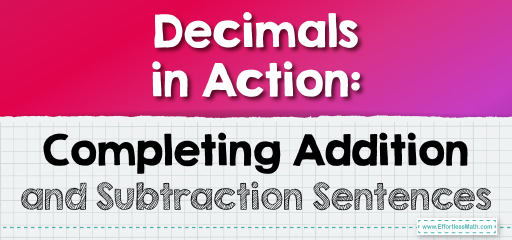# Decimals in Action: Completing Addition and Subtraction Sentences

When working with decimals, we often encounter incomplete sentences or equations that require us to fill in the missing piece. These can be in the form of missing addends, subtrahends, minuends, or even the result itself. Let's explore how to approach and solve these types of problems.### Example 1: Completing an Addition Sentence

$$3.45 + \_ = 7.89$$

Solution Process:

Subtract $$3.45$$ from $$7.89$$.

The missing addend is $$4.44$$.

The Absolute Best Book for 5th Grade Students

### Example 2: Completing a Subtraction Sentence

$$8.23 – \_ = 5.78$$

Solution Process:

Subtract $$5.78$$ from $$8.23$$.

The missing subtrahend is $$2.45$$.

Completing decimal addition or subtraction sentences is a valuable skill that tests your understanding of the operations and your ability to manipulate decimals. By identifying the known values and using addition or subtraction, you can find the missing piece of the equation. This skill not only reinforces your foundational knowledge of decimals but also enhances your problem-solving abilities. With regular practice, you’ll become adept at quickly and accurately completing these sentences, making you a true decimal detective.

### Practice Questions:

1. $$4.56 + \_ = 9.01$$

2. $$7.89 – \_ = 3.45$$

3. $$2.34 + \_ = 6.78$$

4. $$9.01 – \_ = 4.56$$

5. $$5.67 + \_ = 8.90$$

A Perfect Book for Grade 5 Math Word Problems!

1. $$4.45$$

2. $$4.44$$

3. $$4.44$$

4. $$4.45$$

5. $$3.23$$

The Best Math Books for Elementary Students

### What people say about "Decimals in Action: Completing Addition and Subtraction Sentences - Effortless Math: We Help Students Learn to LOVE Mathematics"?

No one replied yet.

X
51% OFF

Limited time only!

Save Over 51%

SAVE $15 It was$29.99 now it is \$14.99